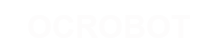### MangoII

• OCROBOT控制器
• 面包板
• 跳线
• 共阳极RGB LED
• 3个220欧姆电阻
• 导线

### 代码

```const int redPin = 3;
const int greenPin = 5;
const int bluePin = 6;```

`Serial.begin(9600);`

```pinMode(redPin, OUTPUT);
pinMode(greenPin, OUTPUT);
pinMode(bluePin, OUTPUT);```

`while (Serial.available() > 0) {`

```int red = Serial.parseInt();
int green = Serial.parseInt();
int blue = Serial.parseInt();```

`if (Serial.read() == '\n') {`

```red = 255 - constrain(red, 0, 255);
green = 255 - constrain(green, 0, 255);
blue = 255 - constrain(blue, 0, 255);```

```analogWrite(redPin, red);
analogWrite(greenPin, green);
analogWrite(bluePin, blue);```

```Serial.print(red, HEX);
Serial.print(green, HEX);
Serial.println(blue, HEX);```
```    }
}
}```

```/*

这个例子演示了串口 parseInt()的功能，它找到以逗号分隔的ascii字符串，把它解析为整形数据作为输入使LED变色。

*/

// LED引脚:
const int redPin = 3;
const int greenPin = 5;
const int bluePin = 6;

void setup() {
//初始化串口通讯
Serial.begin(9600);
// 作为输出:
pinMode(redPin, OUTPUT);
pinMode(greenPin, OUTPUT);
pinMode(bluePin, OUTPUT);

}

void loop() {
// 有串口数据就读取
while (Serial.available() > 0) {

// 在数据流中寻找有效的整数
int red = Serial.parseInt();
// 重复上个过程:
int green = Serial.parseInt();
// 再重复
int blue = Serial.parseInt();

// 寻找换行符，结束符
// 定义常量到0-255 ，反过来
// 如果你用共阴极的LED那就是constrain(color, 0, 255)
red = 255 - constrain(red, 0, 255);
green = 255 - constrain(green, 0, 255);
blue = 255 - constrain(blue, 0, 255);

// 使LED变色:
analogWrite(redPin, red);
analogWrite(greenPin, green);
analogWrite(bluePin, blue);

// 作为十六进制的一个字符串显示在串口
Serial.print(red, HEX);
Serial.print(green, HEX);
Serial.println(blue, HEX);
}
}
}```
learing/examples/readasciistring.txt · 最后更改: 2017/04/07 10:03 (外部编辑)

### 页面工具